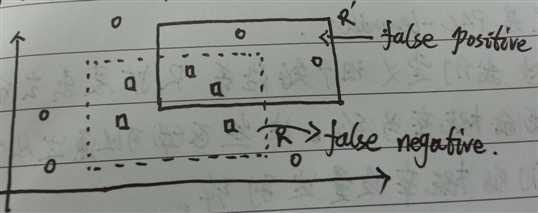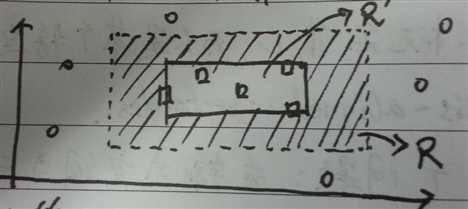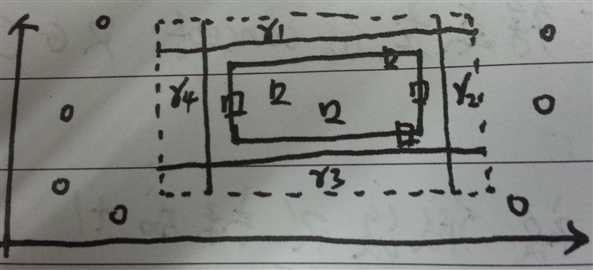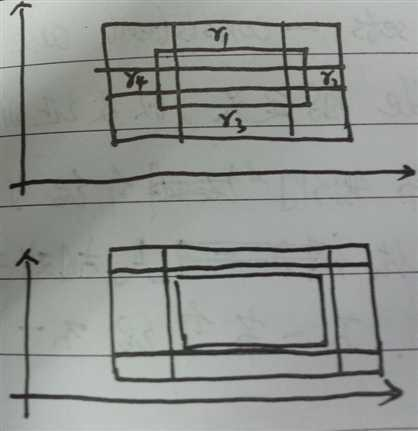Foundations of Machine Learning: The PAC Learning Framework(1)

Foundations of Machine Learning: The PAC Learning Framework(1)

在计算学习理论，probably approximately correct learning(PAC learning)是分析机器学习的一个数学框架。这个框架解决了这样的一些问题：

1. 什么样的概念是能够被有效的学习出来？
2. 要达到一个成功的学习过程，至少要多少样本？

（一）PAC 学习模型

（1）基本概念以及假设。

$\mathcal{X}:$输入空间（input space），所有可能的示例集合。
$\mathcal{Y}:$输出空间（output space），所有可能的标签或者目标值的集合。当$\mathcal{Y}=\{0,1\}$时叫做二分类（binary classification）。
概念（Concept） $c:\mathcal{X} \rightarrow \mathcal{Y}$，是从input space 到output space 的一个映射。
概念集合(Concept Class) $C:$所有$c$的集合。
假设集（Hypothesis  Set） $H:$所有假设$h$的集合。学习者会从这个集合中选出一个假设，做为最后的学习的结果。注意，这与前面的 Concept  class并不一定一致。
一个假设：所有的样本是根据一个未知的分布$D$,以$iid$（独立同分布）的形式采样出来的。

（二）泛化错误以及经验错误。

$$\mathcal{R}(h)= \mathop{Pr}_{x\backsim D}[h(x)\neq c(x)]=E_{x\backsim D}[\mathbb{I}(h(x)\neq c(x))]$$

也就是说在分布$D$已知的情况下，用$h$去近视$c$会产生的真正错误。但通常这个分布$D$是未知的，所以上述泛化错误很难计算，所以用经验错误来估计上述错误。

$$\widehat{\mathcal{R}}(h)=\frac{1}{m}\sum_{i=1}^{m}\mathbb{I}_(h(x)\neq c(x))$$

\begin{eqnarray*}E[\widehat{\mathcal{R}}(h)] &=& E_{s\sim D^m}[\widehat{\mathcal{R}}(h)] \\&=& \frac{1}{m}\sum_{i=1}^{m}E_{s \backsim D^m}[\mathbb{I}(h(x_i \neq c(c_i)))]\\&=& \frac{1}{m}\sum_{i=1}^{m}E_{x\backsim D}[\mathbb{I}(h(x) \neq c(x))]\\&=& E_{x \backsim D}[\mathbb{I}(h(x) \neq c(x))] \\&=& \mathcal{R}(h)\end{eqnarray*}

（三）PAC-学习

$$\mathop{Pr}_{S\sim D^m}[\mathcal{R}(h_S)\leq \varepsilon]\geq 1-\delta,$$

怎样去理解上述定义？对于一个$concept \ class \ C$，如果存在一个算法$\mathcal{A}$，在观察到一定数量的点后，返回一个假设$h$，并且这个假设$h$一很高的概率$(1-\delta)$保证$h$与$concept \ c$的接近度小于$\epsilon$，那么这个$concept \ class \ C$就是PAC-learnable。

关于PAC框架的定义，必须注意三点：

1. 它是一个distribution-free model，也就是说没有对分布作任何假设。
2. 测试样本和训练样本必须来自同分布。
3. PAC解决的是某一概念集C，而不是某个特定的概念。

（四）例子：与坐标轴对齐的矩形。

我们来学习这样一个问题：输入空间为二维平面$R^2$，在这个二维平面上，只有某个与坐标轴对齐的矩阵内的点的label为+1，其他label为-1。我们来证明该问题是PAC-learnable。首先，把这个问题抽象成一个数学模型。其中，$\mathcal{A}=R^2,\mathcal{Y}=\{+1,-1\}$，概念集合 $C=\{$所有与坐标轴对齐的矩形内的点为+1$\}$，假设集 $H=\{$所有与坐标轴对齐的矩形内的点为+1$\}$。1. false positive: 本来为-1的被假设$R‘$标成+1，称为假阳性。
2. false negative: 本来为+1的被假设$R‘$标成-1，称为假阴性。所以，我们假设 $\mathop{Pr}[R] > \epsilon$。我们定义沿着$R$扩展出去的矩阵区域为$r_1,r_2,r_3,r_4$，并且每个区域的概率为$\epsilon /4$。这些区域可以通过从空矩阵开始增长，直到它们的概率质量达到$\epsilon / 4$。如图三所示：\begin{align}\mathcal{R}[R_s] &= \mathop{Pr}[shaded \ area]&\leq \mathop{Pr}[r_1] + \mathop{Pr}[r_1] + \mathop{Pr}[r_3] + \mathop{Pr}[r_4]&= \epsilon\end{align}$$\mathop{Pr}[r_1]+\mathop{Pr}[r_2]+\mathop{Pr}[r_3]+\mathop{Pr}[r_4]=\epsilon$$

$$\mathop{Pr}_{s \backsim D^m}[\mathcal{R} \leq \epsilon] > \mathop{Pr}_{s \backsim D^m}[R_s \ intersect \ with \ r_1,r_2 ,r_3,r_4 \ at \ the \ same \ time]$$

\begin{eqnarray}& & \mathop{Pr}_{s \backsim D^m}[\mathcal{R}[R_s] > \epsilon] \nonumber\\&\leq& 1 - \mathop{Pr}_{s\backsim D^m}[R_s \ intersect \ with \ r_1,r_2 ,r_3,r_4 \ at \ the \ same \ time]\nonumber\\ &=& \mathop{Pr}_{s\backsim D^m}[R_s \ non-intersect \ with \ r_1,r_2,r_3,r_4 \ at \ least \ one]\nonumber\\ &=& \mathop{Pr}_{s\backsim D^m}[\bigcup_{i=1}^{4}\{R_s\bigcap r_i=\varnothing\}] \ \ \ \ \ \ (Use \ Union \ Bound)\nonumber\\ &\leq& \sum_{i=1}^{4}\mathop{Pr}_{s\backsim D^m}[\{R_s \bigcap r_i = \varnothing\}]\\ &\leq& 4(1-\epsilon/4)^m\label{equ:1}\\ &\leq& 4exp(-m\epsilon/4)\end{eqnarray}

$$4exp(-m\epsilon/4)\leq \delta \Longleftrightarrow m \geq \frac{4}{\epsilon}log\frac{4}{\delta}$$

Foundations of Machine Learning: The PAC Learning Framework(1),布布扣,bubuko.com

Foundations of Machine Learning: The PAC Learning Framework(1)

(0)
(0)